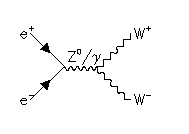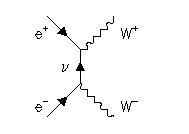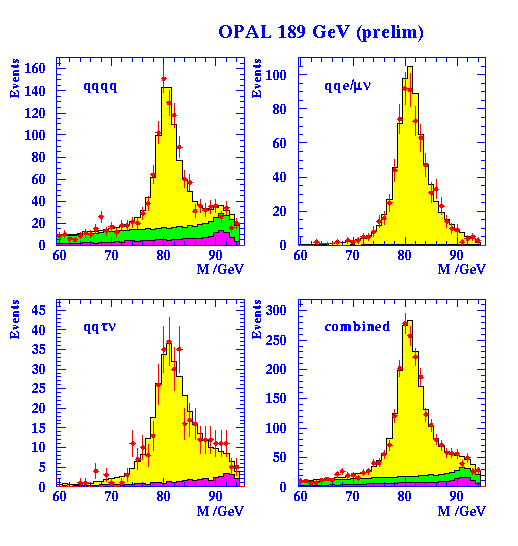# Measurement of the W boson mass

In 1996 the beam energy of LEP was increased so that pairs of weak W bosons may be formed. The first runs in 1996 were at a centre-of-mass energy of 161 GeV, and progressively increased to just over 200 GeV. The relevant Feynman diagrams for W+W- production are these:One of the important measurements at LEP 2 is the precise measurement of the mass of the W, and this is under study at Cambridge. The mass of the W can be predicted indirectly from the existing measurements of the Z at LEP1, with an accuracy of about 0.03 GeV. A measurement at LEP 2 to this precision of better will therefore form an important test of the validity of the Standard Model.

The W bosons can either decay to quark + antiquark, or to charged lepton + neutrino. If both Ws decay to quarks, the event is seen to have (at least) four jets of hadrons, as in this event.

In the case where one W decays to quarks and the other to leptons, one typically sees two jets together with an isolated high energy lepton, as in this event where a high energy electron is marked in red. The missing momentum, which can be associated with the neutrino, is indicated by the arrow.

The mass of the W boson may be measured by directly reconstructing the invariant mass of pairs of jets. However, the selection of events is not completely free from background (from Z decays, or from incorrect pairings of jets in WW events). The plot below shows an example of the sort of mass peak we are able to reconstruct in OPAL using four-jet and events containing leptonic W decays. Based on our present results, we expect to be able to achieve a precision of 0.03 GeV on the W mass by the end of LEP 2, after combining results from all the LEP experiments.